### Steam Drum Level

Steam drum level measurement with a differential pressure transmitter can be a tricky business when the pressure is higher than for "low" pressure steam. What happens is that as the temperature rises, the density of water drops while at the same time that of steam rises. To compound the problem, the wet leg temperature is not well defined and its density is a third variable.

A technical way around the wet leg problem is to use the following level capture apparatus.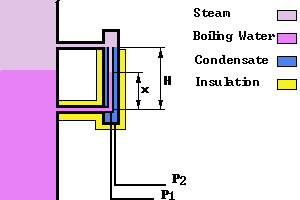. The constant condensation in the top connection maintains a constant influx of hot water at equilibrium with the steam. This maintains the heat and ensures both wet leg and measurement sections are at the same temperature (that of the water in the steam drum), below the apparatus, the two impulse lines are in close contact and therefore at the same temperature. Whatever the density of the water is, it is the same in both legs and cancels out in the differential measurement.

Within the apparatus itself, the dilemma remains. The simplest way is to use a correlation to provide the steam and the water densities as a function of the absolute pressure in the boiler.

Looking at figure 1, we see that the differential pressure, P2 - P1 is: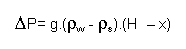where g is the gravitational acceleration constant: 9.807 m/s2, and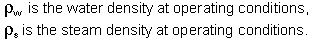Furthermore we assume the transmitter is calibrated with cold water which has a density of: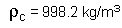We note that when the level is 100%, the differential pressure is 0, regardless of whether the water is hot or cold. When the level is 0%, the differential pressure is maximum although this maximum value depends on the pressure in the steam drum.

Now, assuming the transmitter has a reversed output, Lraw, calibrated with cold water, so that when the level increases so does the analog signal, then it is possible to express the level, L, in % as a function of the densities of the water (both hot and cold) and of the steam: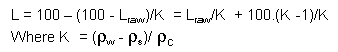To do so I have calculated three fourth degree polynomials which predict the density of steam, water, and the correction term K as a function of pressure. Those polynomials have been derived using the ASME steam tables and using least square regression to obtain the best fit.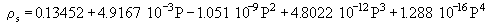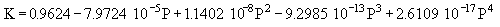The densities are in kg/m3 and the pressure is in kPa absolute.

For the steam density the relative error is less than +/-1% for all pressures between 500 and 15000 kPa(abs). The relative error becomes larger at low pressures being 6% at 100 kPa(abs)

For the water density and for K the relative error is +/-1% or less from 0 kPa(abs) to 15000kPa(abs)

This relatively simple formula will correct the dP measurement and indicate the correct level from stone cold to over 340Ḟ C.